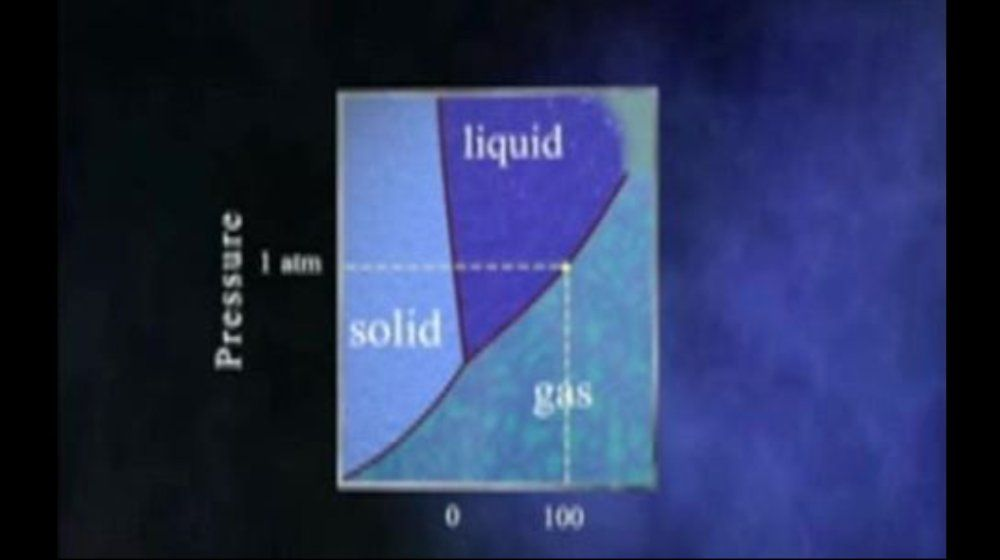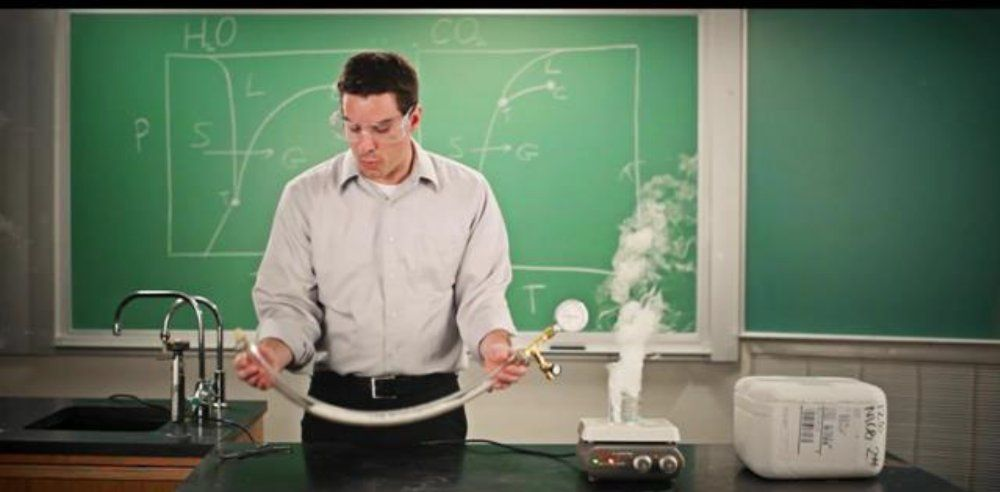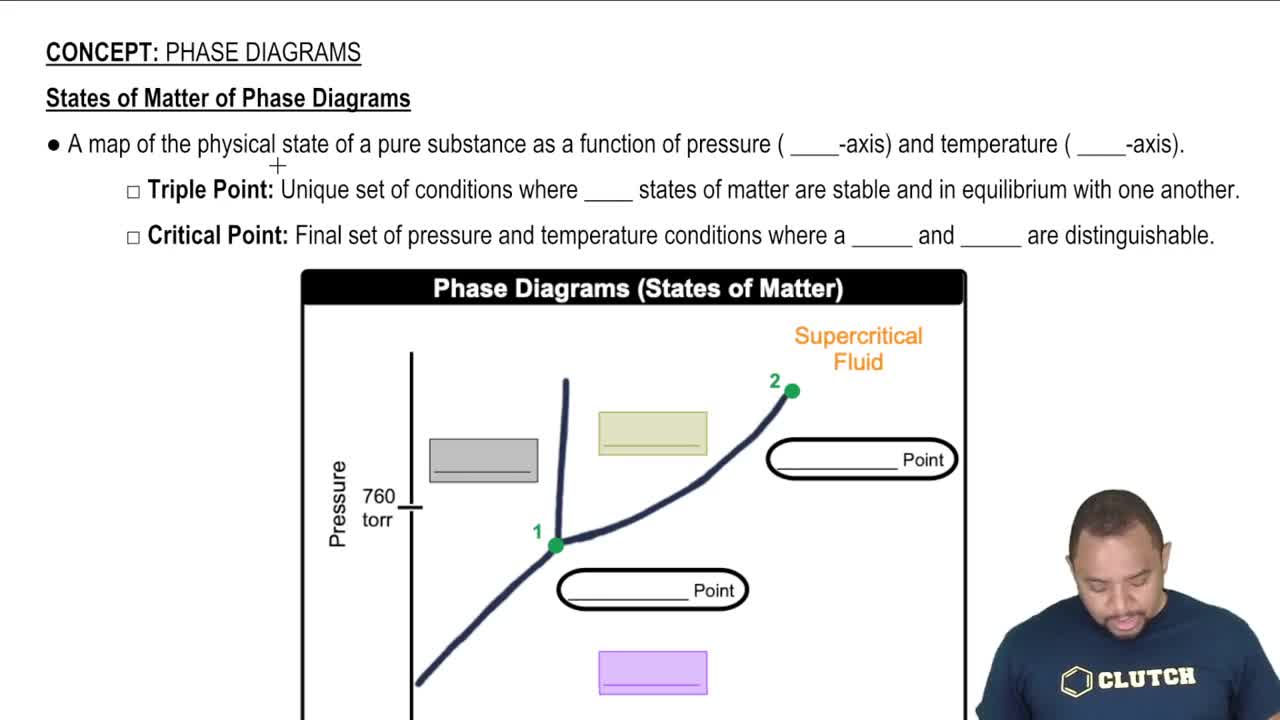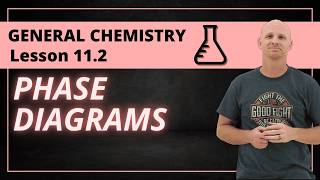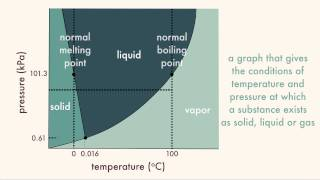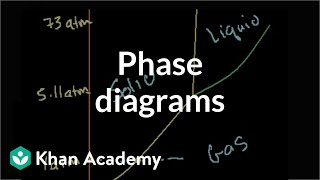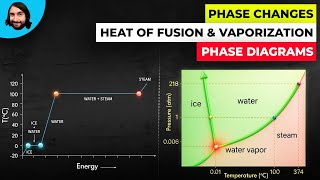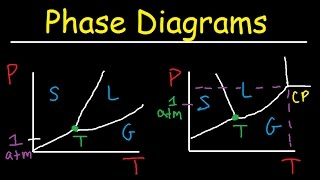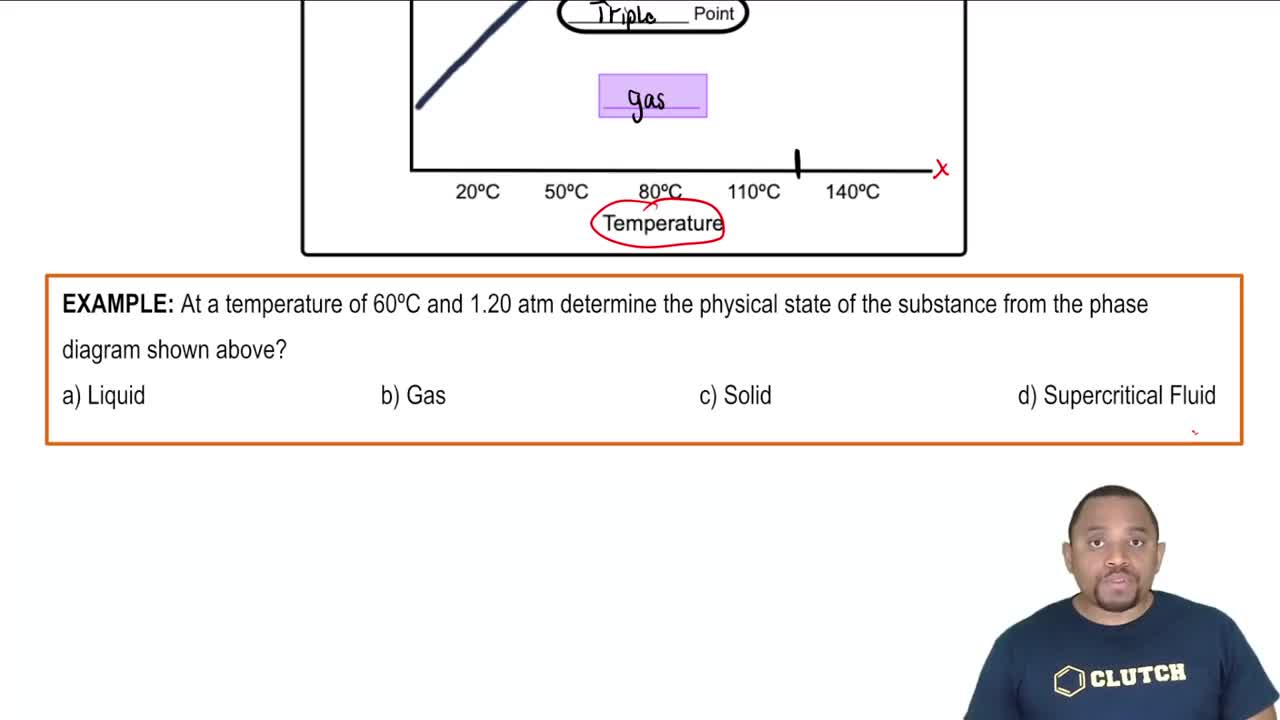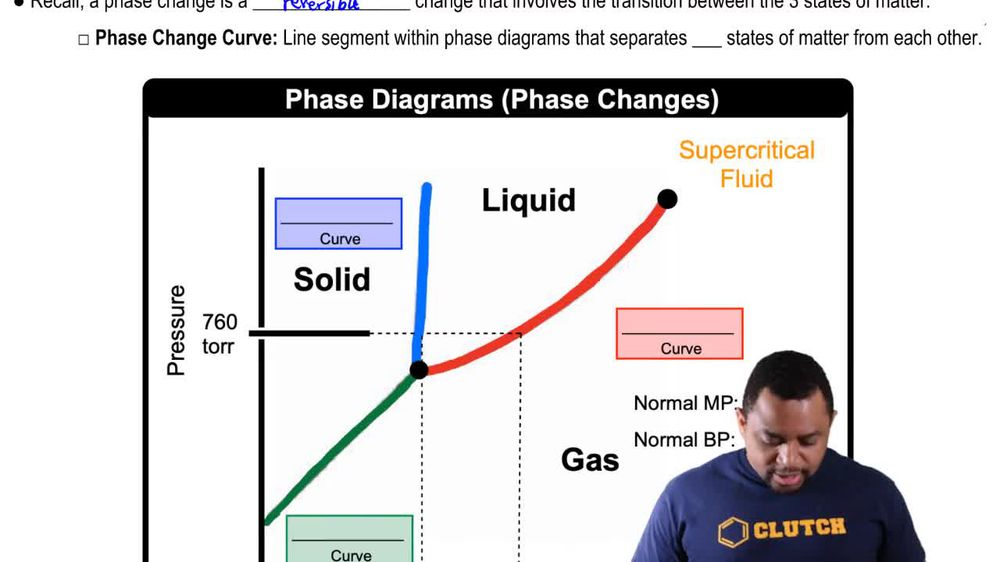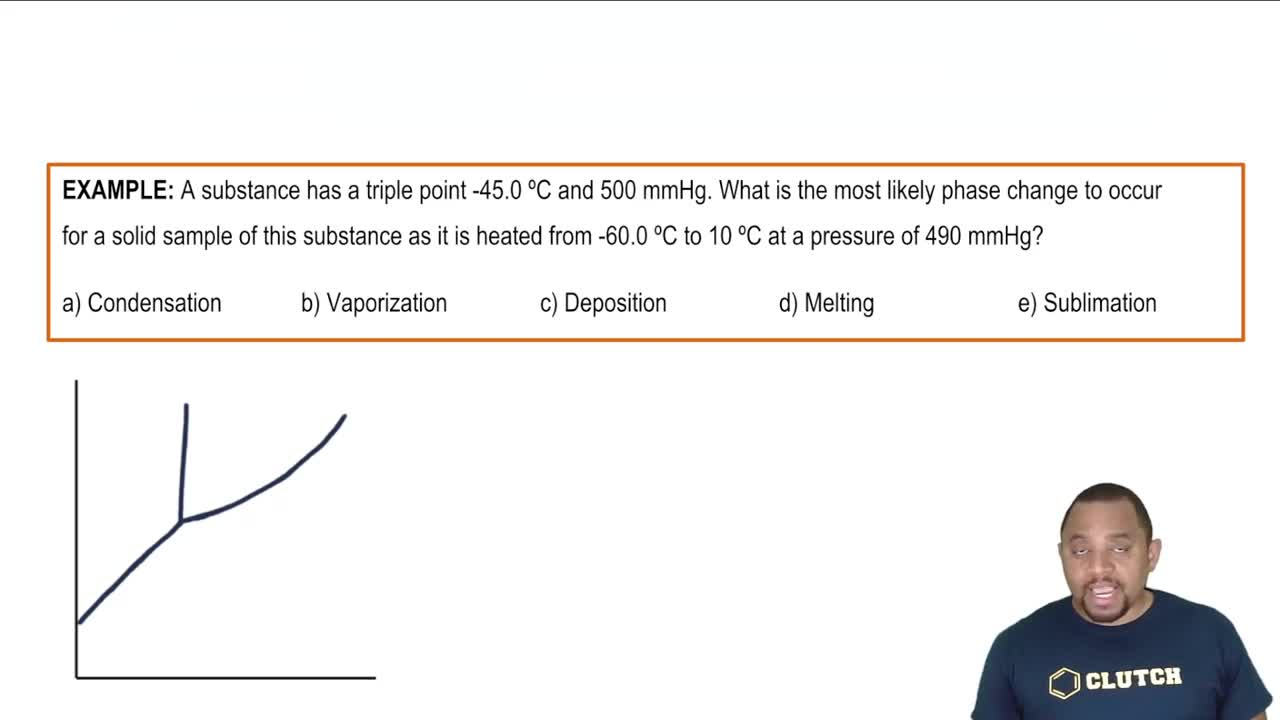Start typing, then use the up and down arrows to select an option from the list.
1. 13. Liquids, Solids & Intermolecular Forces2. Phase Diagrams# Phase Diagrams Example 2

by Jules Bruno
114 views
0
a substance has a triple point of negative 45 degrees Celsius and the pressure of 500 millimeters of mercury. What is the most likely phase change to occur for a solid sample of the substance as it's heated from negative 60 degrees Celsius to 10 degrees Celsius at a pressure of 490 millimeters of mercury. Alright, so our triple point we're going to say is right here. And at this point we have a pressure of 500 millimeters per mercury melon using mercury and a temperature of negative 45 degrees Celsius. Remember here we have our solid or liquid and our gas, They say that our pressure now is 490, so it's gonna be lower than this triple point. So let's say 4 90s around here. Right. So that would mean that would fall somewhere between either being a solid or a gas, were no longer able to become a liquid since the pressure has decreased And they're saying we're going from negative 60°. So negative 60, we can say is over here and we're going to 10°C, which is somewhere over here. So, if we can just look at this, we can see that we're going from somewhere over here and we're transitioning over to the gashes phase. So we're going from a solid to a gas that would mean that we're undergoing sublimation. So here option e would be the correct answer